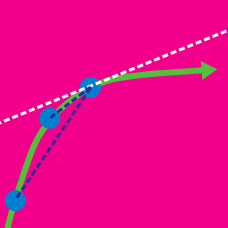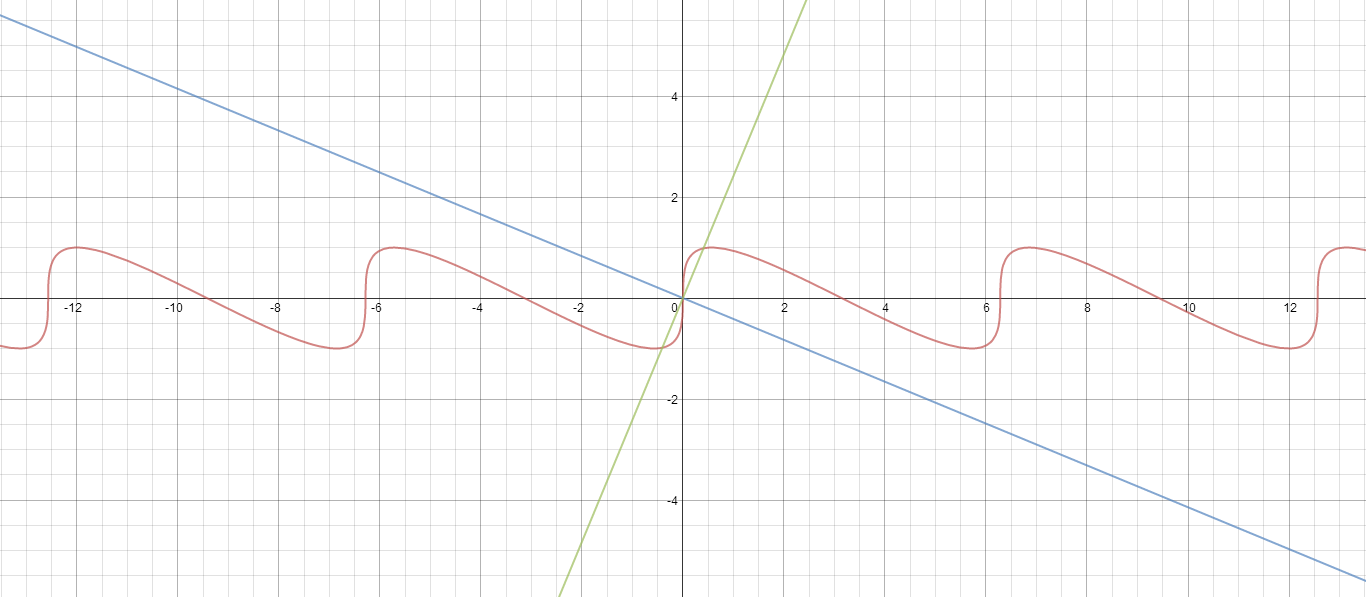Calculus

# Differentiability: Level 4 Challenges

$\lambda=\left( \frac{f(5102)-f(2015)}{f'(c)} \right) \left( \frac{f^2(2015)+f^2(5102)+f(2015)f(5102)}{f^2(c)} \right)$

Let $f:[2015,5102] \rightarrow [0,\infty)$be any continuous and differentiable function. Find the value of $\lambda$, such that there exists some $c\in [2015,5102]$ which satisfies the equation above.

$f(x) = \prod_{i=1}^{\infty} \frac{1+ 2\cos\left( \frac{2x}{3^i} \right)}{3}$

Let $f(x)$ denote an function as described above. The number of points where $|xf(x)| + | |x-2|-1|$ is non-differentiable in $x \in (0,3\pi)$ is $k$, find $k^2$.$(\alpha_1, \beta_1), \ (\alpha_2, \beta_2), \ \cdots \ , \ (\alpha_n, \beta_n)$ are the points on the curve $S \ : \ y=\sin (x+y) \ \ \forall x \in [-4\pi, \ 4\pi]$
such that the tangents at these points to $S$ are perpendicular to the line $l \ : \ (\sqrt {2}-1)x+y=0.$

Find the value of $\left\lfloor \displaystyle\sum_{k=1}^{n} |\alpha_k|-|\beta_k|\right\rfloor.$

Details and Assumptions

• $\lfloor{\cdots}\rfloor$ denotes the floor function (greatest integer function).

• Even though in the problem, a plural form of points is given, there may only be one such point that exists.

What is the largest possible number of integers $n$ such that for some fixed polynomial $f(x)$ of degree $3$ with integer coefficients, $f(n)=n^{1000}$?

Let $f(x)$ be a non-constant thrice differentiable function defined on real numbers such that $f(x)=f(6-x)$ and $f'(0)=0=f'(2)=f'(5)$. Find the minimum number of values of $p \in [0,6]$ which satisfy the equation $(f''(p))^2+f'(p)f'''(p)=0$ Details and Assumptions:

• $f'(p)=\left( \frac{df(x)}{dx} \right)_{x=p}$

• $f''(p)=\left( \frac{d^2f(x)}{dx^2} \right)_{x=p}$

• $f'''(p)=\left( \frac{d^3f(x)}{dx^3} \right)_{x=p}$

×